# Inelastic Collision Formula

Inelastic Collision Formula

An inelastic collision is any collision between objects in which some energy is lost. A special case of this is sometimes called the "perfectly" inelastic collision. In a perfectly inelastic collision, two objects collide and stick together. The momentum of the objects before the collision is conserved, but the total energy is not conserved. The final velocity of the combined objects depends on the masses and velocities of the two objects that collided. The units for the initial and final velocities are m/s, and the unit for mass is kg.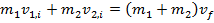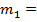mass of a first object (kg)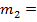mass of a second object (kg)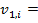initial velocity of the first object (m/s)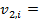initial velocity of the second object (m/s)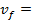final velocity of the combined objects (m/s)

Inelastic Collision Formula Questions:

1) A man shoots a paintball at an old can on a fencepost. The paintball pellet has a mass of 0.200 g, and the can has a mass of 15.0 g. The paintball hits the can at a velocity of 90.0 m/s. If the full mass of the paintball sticks to the can and knocks it off the post, what is the final velocity of the combined paintball and can?

Answer: The final velocity can be found for the combined paintball and can by rearranging the formula: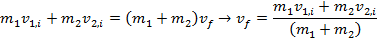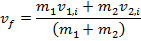The mass of the paintball is 0.200 g, which is equal to 0.000200 kg, and the mass of the can is 15.0 g, which is equal to 0.015 kg. The initial velocity of the paintball is 90.0 m/s. The can starts at rest, so its initial velocity is 0.0 m/s.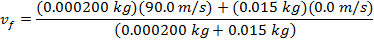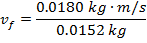1.18 m/s

After the collision, the velocity of the paintball and can together is 1.18 m/s.

2) A young boy is sledding down a very slippery snow-covered hill. He has a mass of 20.0 kg, and he is sliding down the hill at a velocity of 5.00 m/s. The boy's older brother has a mass of 30.0 kg, and is farther down the hill. He is moving slower with a velocity of 2.00 m/s. The younger boy catches up to his brother, and grabs hold of him and his sled. The two of them continue down the hill as one unit. What is the resulting velocity of the boys?

Answer: The final velocity can be found for the two boys by rearranging the formula: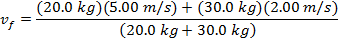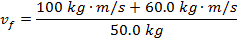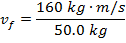3.20 m/s

After the boys collide, their combined velocity is 3.20 m/s.

 Related Links: Reaction Rates and Collisions Quiz Momentum Formula Elastic Collision Formula